# 移动端arm cpu优化学习笔记第2弹--常量阶时间复杂度中值滤波

Median Filtering in Constant Time

Ldpe2G/ArmNeonOptimization

1、一般中值滤波的实现

median_filter(const float  *input,
const int     height,
const int     width,
float        *output) {

int out_idx = 0;
for (int h = 0; h < height; ++h) {
const int h_lower_bound = std::max(0, h - radius);
const int h_upper_bound = std::min(height - 1, h + radius);
const int h_interval = h_upper_bound - h_lower_bound + 1;

for (int w = 0; w < width; ++w) {
const int w_left_bound = std::max(0, w - radius);
const int w_right_bound = std::min(width - 1, w + radius);
const int arr_len = h_interval * (w_right_bound - w_left_bound + 1);

int idx = 0;
for (int i = h_lower_bound; i <= h_upper_bound; ++i) {
const int h_idx = i * width;
for (int j = w_left_bound; j <= w_right_bound; ++j) {
m_cache[idx ++] = input[h_idx + j];
}
}

sortArr(m_cache.data(), arr_len);
output[out_idx ++] = m_cache[arr_len / 2];
}
}
}


static void sortArr(float *arr, int len) {
const int middle = len / 2;
for (int i = 0; i <= middle; ++i) {
float min = arr[i];
int min_idx = i;
for (int j = i + 1; j < len; ++j) {
if (min > arr[j]) {
min_idx = j;
min = arr[j];
}
}
// swap idx i and min_idx
float tmp = arr[min_idx];
arr[min_idx] = arr[i];
arr[i] = tmp;
}
}


132, 45, 8, 1, 9, 100, 34

1, 8, 9, 34, 45, 100, 132

132 , 45, 8, 1 , 9, 100, 34 -> 1, 45, 8, 132, 9, 100, 34

1, 45 , 8 , 132, 9, 100, 34 -> 1, 8, 45, 132, 9, 100, 34

1, 8, 45 , 132, 9 , 100, 34 -> 1, 8, 9, 132, 45, 100, 34

1, 8, 9, 132 , 45, 100, 34 -> 1, 8, 9, 34, 45, 100, 132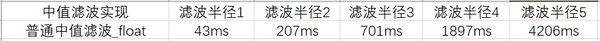2、第一版优化，float数据类型改uint16_t

https://github.com/Ldpe2G/ArmNeonOptimization/blob/master/ConstantTimeMedianFilter/src/normal_median_filter_uint16.cpp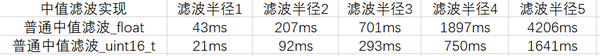3，第二版优化，简单利用并行计算指令

#if defined(USE_NEON_INTRINSIC) && defined(__ARM_NEON)
int neon_arr_len = h_interval * (w_end - w_start + 1) * 8;
for (int w = w_second_loop_start; w < remain_start; w += 8) {
const int w_left_bound = std::max(0, w + w_start);
const int w_right_bound = std::min(width - 1, w + w_end);

int idx = 0;
for (int i = h_lower_bound; i <= h_upper_bound; ++i) {
const int h_idx = i * width;
for (int j = w_left_bound; j <= w_right_bound; ++j) {
for (int q = 0; q < 8; ++q) {
m_cache[idx ++] = input[h_idx + j + q];
}
}
}

sortC4ArrNeon(m_cache.data(), neon_arr_len);
for (int i = 0; i < 8; ++i) {
m_out_buffer[out_idx ++] = m_cache[(neon_arr_len / 8 / 2) * 8 + i];
}
}
#endif


https://github.com/Ldpe2G/ArmNeonOptimization/blob/master/ConstantTimeMedianFilter/src/normal_median_filter_uint16.cpp#L102

#if defined(USE_NEON_INTRINSIC) && defined(__ARM_NEON)
static void sortC4ArrNeon(uint16_t *arr, int len) {
const int actual_len = len / 8;
const int middle = actual_len / 2;
uint16_t *arr_ptr = arr;
for (int i = 0; i <= middle; ++i) {
uint16x8_t  min = vld1q_u16(arr_ptr);
uint16x8_t   min_idx = vdupq_n_u16(i);

uint16_t *inner_arr_ptr = arr_ptr + 8;
for (int j = i + 1; j < actual_len; ++j) {
uint16x8_t curr =  vld1q_u16(inner_arr_ptr);
uint16x8_t   curr_idx = vdupq_n_u16(j);
uint16x8_t  if_greater_than = vcgtq_u16(min, curr);
min     = vbslq_u16(if_greater_than, curr, min);
min_idx = vbslq_u16(if_greater_than, curr_idx, min_idx);
inner_arr_ptr += 8;
}
// swap idx i and min_idx
for (int q = 0; q < 8; ++q) {
float tmp = arr[min_idx[q] * 8 + q];
arr[min_idx[q] * 8 + q] = arr[i * 8 + q];
arr[i * 8 + q] = tmp;
}
arr_ptr += 8;
}
}
#endif // __ARM_NEON


vcgtq 表示将第一个参数内的数组元素与第二个参数对应元素比较，如果第一个数组的元素，大于等于对应第二个数组的对应元素，则结果对应位置会置为1，否则为0。

vbslq 指令有三个输入，第一个输入可以看做是判断条件，如果第一个输入的元素位置是1则结果的对应的位置就取第二个输入的对应位置，否则从第三个输入对应位置取值。其实这和mxnet的where操作子很像。

ok，我们接着来看下这版的耗时：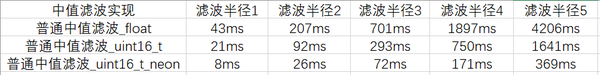4，第三版优化，算法上的改进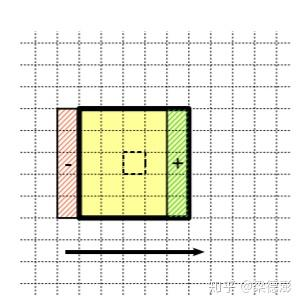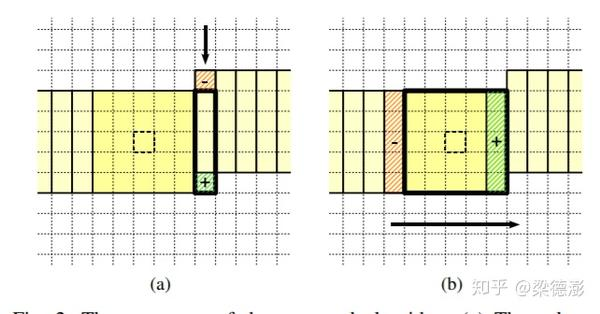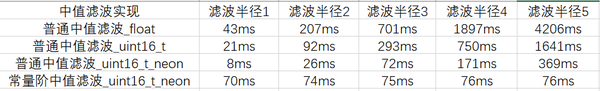A coarse-to-fine algorithm for fast median filtering of image data with a huge number of levels